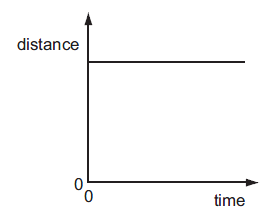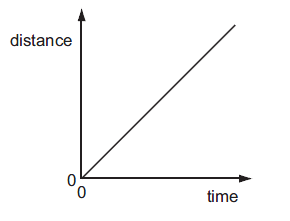# Draw the shape of distance- time graph for:a. A man, waiting for a bus, standing at one point, on a bus stand. b. A man, walking on a level, straight and narrow Road, with a constant speed.

a.

Here the person is at rest. So, distance will be constant.

The graph will be a straight line parallel to x axisb.

Here the person is moving with constant speed. Speed is slope of distance-time graph.

Here the graph will be straight line with constant slopeUpdated on: 10-Oct-2022

50 Views